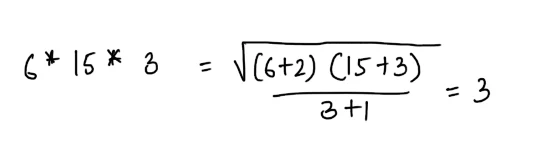New Student User - Use Code HELLO

# Mixed Aptitude Quiz for RBI Grade B Exam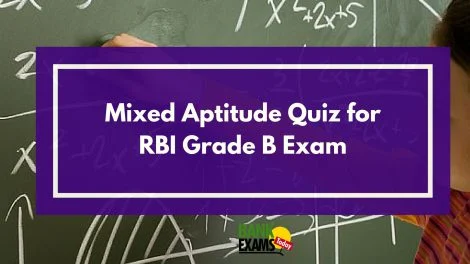#### Ques 1.

A man has some oranges , out of which 2% are rotten.He sells 95%of the remaining oranges. Now he is left with 49 oranges .How many oranges had he in all ?

(a) 800
(b) 1000
(c) 600
(d) 9000
Ans 1. Let the number  of oranges the man had be x.#### Ques 2.

What percentage error will be made in the calculated volume of a sphere, if the measured value of the radius is 1 1/2% too large ?
(a) 4.6
(b) 5.2
(c) 1.1
(d) 3.6
Ans 2.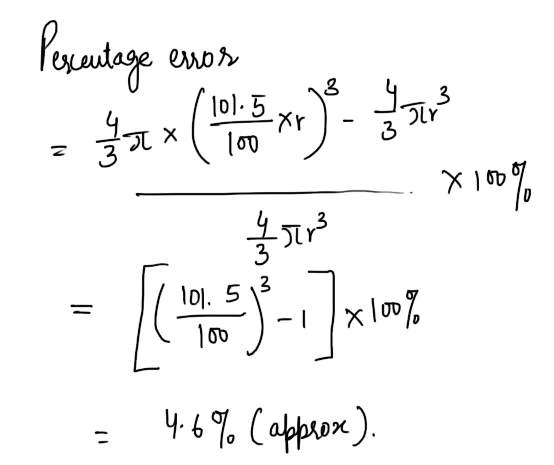#### Ques 3.

How many numbers between 100 and 600 are divisible by 5 and 6 both ?
(a) 60
(b) 17
(c) 22
(d) 38
Ans 3. Numbers that are divisible by 5 and 6 both  must be divisible by 30.( 5 and 6 are coprime) .
.  . The desired numbers are : 120, 150, 180 ......600 which are 17 in number .

#### Ques 4.

If a man walks at the rate of 5km/hr, he misses a train by only 7 minutes . However, if he walks at the rate of 6/km/hr, he reaches the station 5 minutes before arrival of the train. The distance covered by him to reach the station is
(a) 5
(b) 6
(c) 7
(d) 8
Ans 4. Let the distance covered by  the man be x km.#### Ques 5.

A merchant in Bombay buys some radio sets from Calcutta , the catalogue Price of which is Rs 600 per set. The trade discount allowed to him by the Calcutta company is 15% on catalogue price. The freight and other charges amount 10 2 1/2% of the catalogue Price/ The Bombay merchant sells each set at 10% discounts on his list price of Rs. 750 thus making a not profit of Rs 1350 . The number of ratio sets bought is
(a) 7
(b) 8
(c) 9
(d) 11
Ans 5.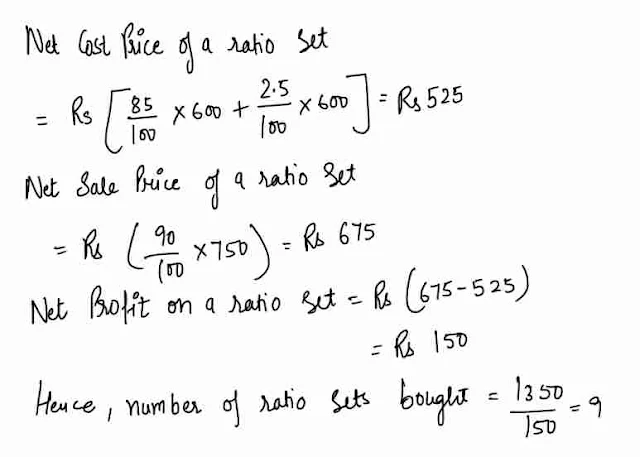#### Ques 6.

The cost of gravelling at the rate of 10 p per m2 the area of a parallelogram whose adjacent sides are 13 m and 140 m and one of the diagonals is 150 m is
(a) Rs 1880
(b) Rs 1680
(c) Rs 1061
(d) Rs 1860
Ans 6.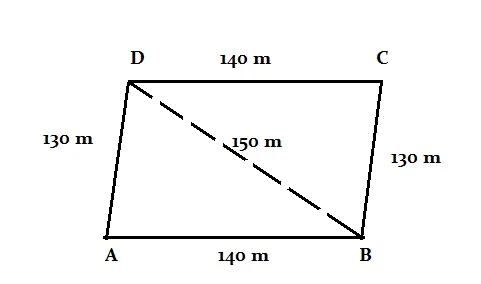#### Ques 7.

The successive discounts of 20% and 15% are equal to a single discount of
(a) 35%
(b) 32 %
(c) 22%
(d) 5%
Ans 7.#### Ques 8.

In the product 459 x 46 x 28* x 484, the digit in the unit's place is 2. The digit to come in place of * is
(a) 3
(b) 5
(c) 7
(d) 1
Ans 8.  9 x 6 x * x 4 must give 2 in the unit's place.
or  6 x * must give 2 in the unit's place .
=>  * = 2, 7

#### Ques 9.

In an examination 35% candidates failed in one subject and 42% failed in another subject while 15% failed in both the subjects .If 2500 candidates appeared at the examination how many passed in both the subjects ?
(a) 950
(b) 1175
(c) 2125
(d) None of these
Ans 9. Percentage of candidates who that failed in at least one of the subjects
= ( 35- 15) + 15 ( 42 - 15)  = 62%
. . Percentage of candidates  who passed  in both the subjects =100 - 62 = 38%
Hence, number of candidates who passed
in both  the subjects = 38/100 x 2500 = 950.

#### Ques 10.

If x * y * z = /(x + 2) ( y + 3) / z + 1 , the value of 6 * 15 * 3 is
(a) 2
(b) 3
(c) 4
(d) None of these
Ans 10.